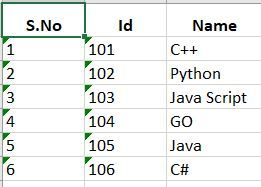# Writing to Excel Sheet Using EPPlus in C#

EPPlus is a very helpful open-source 3rd party DLL for writing data to excel. EPPlus supports multiple properties of spreadsheets like cell ranges, cell styling, charts, pictures, shapes, comments, tables, protection, encryption, pivot tables, data validation, conditional formatting, formula calculation, etc.

First of all install EPPlus using Packet Manager console by writing the following command:

```Install-Package EPPlus
```

Let’s see how to create and write to an excel-sheet using C#.

 `using` `System; ` `using` `System.IO; ` `// The following to two namespace contains ` `// the functions for manipulating the ` `// Excel file  ` `using` `OfficeOpenXml; ` `using` `OfficeOpenXml.Style; ` ` `  `class` `Program ` `{ ` `    ``static` `void` `Main(``string``[] args) ` `    ``{ ` `        ``var` `Articles = ``new``[] ` `        ``{ ` `                ``new` `{ ` `                    ``Id = ``"101"``, Name = ``"C++"` `                ``}, ` `                ``new` `{ ` `                    ``Id = ``"102"``, Name = ``"Python"` `                ``}, ` `                ``new` `{ ` `                    ``Id = ``"103"``, Name = ``"Java Script"` `                ``}, ` `                ``new` `{ ` `                    ``Id = ``"104"``, Name = ``"GO"` `                ``}, ` `                ``new` `{ ` `                    ``Id = ``"105"``, Name = ``"Java"` `                ``}, ` `                ``new` `{ ` `                    ``Id = ``"106"``, Name = ``"C#"` `                ``} ` `            ``}; ` ` `  `        ``// Creating an instance ` `        ``// of ExcelPackage ` `        ``ExcelPackage excel = ``new` `ExcelPackage(); ` ` `  `        ``// name of the sheet ` `        ``var` `workSheet = excel.Workbook.Worksheets.Add(``"Sheet1"``); ` `         `  `        ``// setting the properties ` `        ``// of the work sheet  ` `        ``workSheet.TabColor = System.Drawing.Color.Black; ` `        ``workSheet.DefaultRowHeight = 12; ` ` `  `        ``// Setting the properties ` `        ``// of the first row ` `        ``workSheet.Row(1).Height = 20; ` `        ``workSheet.Row(1).Style.HorizontalAlignment = ExcelHorizontalAlignment.Center; ` `        ``workSheet.Row(1).Style.Font.Bold = ``true``; ` `     `  `        ``// Header of the Excel sheet ` `        ``workSheet.Cells[1, 1].Value = ``"S.No"``; ` `        ``workSheet.Cells[1, 2].Value = ``"Id"``; ` `        ``workSheet.Cells[1, 3].Value = ``"Name"``; ` ` `  `        ``// Inserting the article data into excel ` `        ``// sheet by using the for each loop ` `        ``// As we have values to the first row  ` `        ``// we will start with second row ` `        ``int` `recordIndex = 2; ` `         `  `        ``foreach` `(``var` `article ``in` `Articles) ` `        ``{ ` `            ``workSheet.Cells[recordIndex, 1].Value = (recordIndex - 1).ToString(); ` `            ``workSheet.Cells[recordIndex, 2].Value = article.Id; ` `            ``workSheet.Cells[recordIndex, 3].Value = article.Name; ` `            ``recordIndex++; ` `        ``} ` `         `  `        ``// By default, the column width is not  ` `        ``// set to auto fit for the content ` `        ``// of the range, so we are using ` `        ``// AutoFit() method here.  ` `        ``workSheet.Column(1).AutoFit(); ` `        ``workSheet.Column(2).AutoFit(); ` `        ``workSheet.Column(3).AutoFit(); ` ` `  `        ``// file name with .xlsx extension  ` `        ``string` `p_strPath = ``"H:\\geeksforgeeks.xlsx"``; ` `         `  `        ``if` `(File.Exists(p_strPath)) ` `            ``File.Delete(p_strPath); ` ` `  `        ``// Create excel file on physical disk  ` `        ``FileStream objFileStrm = File.Create(p_strPath); ` `        ``objFileStrm.Close(); ` ` `  `        ``// Write content to excel file  ` `        ``File.WriteAllBytes(p_strPath, excel.GetAsByteArray()); ` `        ``//Close Excel package ` `        ``excel.Dispose(); ` `        ``Console.ReadKey(); ` ` `  `    ``} ` `} `

Output:In this program, we are taking static values for the Articles data but in real-time, we can use database call and foreach loop for iteration of each record.

My Personal Notes arrow_drop_upCheck out this Author's contributed articles.

If you like GeeksforGeeks and would like to contribute, you can also write an article using contribute.geeksforgeeks.org or mail your article to contribute@geeksforgeeks.org. See your article appearing on the GeeksforGeeks main page and help other Geeks.

Please Improve this article if you find anything incorrect by clicking on the "Improve Article" button below.

Improved By : vibs2006

Article Tags :

1

Please write to us at contribute@geeksforgeeks.org to report any issue with the above content.